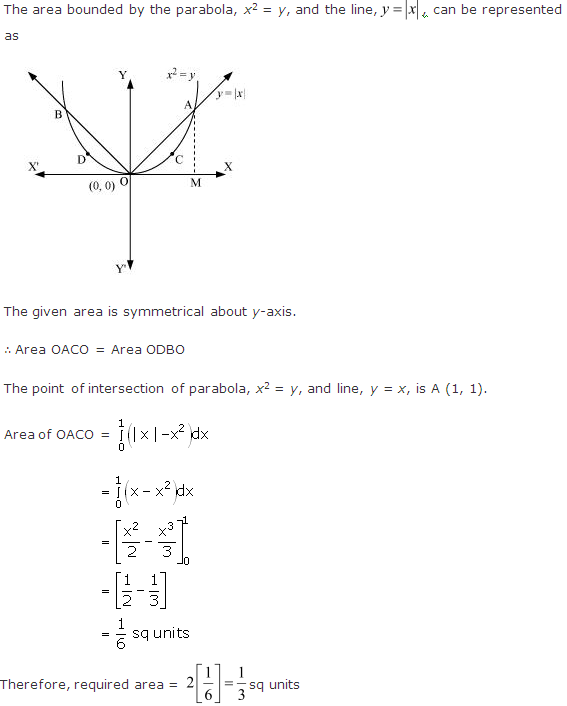# NCERT Solutions for Class 12-science Maths Chapter 8 - Applications of Integrals

Page / Exercise

## Chapter 8 - Applications of Integrals Exercise Ex. 8.1

Solution 1Solution 2Solution 3Solution 4Solution 5Solution 6Solution 7Solution 8Solution 9Solution 10Solution 11Solution 12Solution 13## Chapter 8 - Applications of Integrals Exercise Ex. 8.2

Solution 1Solution 2Solution 3Solution 4Solution 5Solution 6Solution 7## Chapter 8 - Applications of Integrals Exercise Misc. Ex.

Solution 1Solution 2Solution 3Solution 4Solution 5Solution 6Solution 7Solution 8Solution 9Solution 10Solution 11Solution 12Solution 13Solution 14Solution 15Solution 16Required area = A(DCOD) + A(OAB)Since the area is positiveThus, the total area =units

Hence, the required area of the region isunits.

Solution 17Required area = A(DCOD) + A(OAB)Since the area is positiveThus, the total area =units

Hence, the required area of the region isunits.

Solution 18Solution 19### STUDY RESOURCES

REGISTERED OFFICE : First Floor, Empire Complex, 414 Senapati Bapat Marg, Lower Parel, Mumbai - 400013, Maharashtra India.